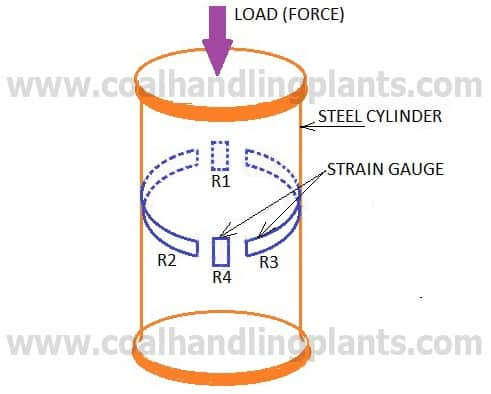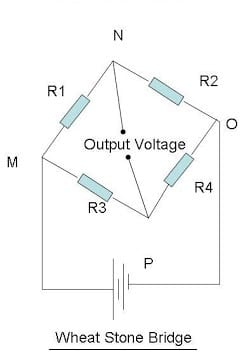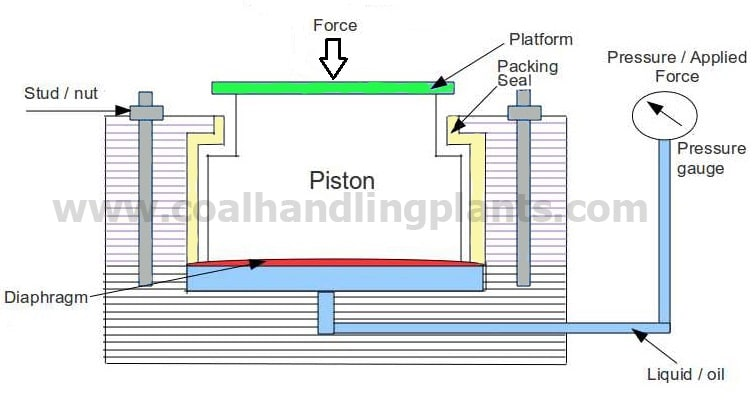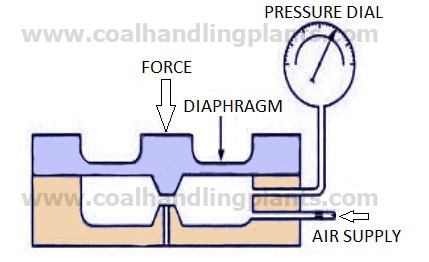A load cell is a transducer which converts force into a measurable electrical output.

1. According to the type of output signal generated

2. According to the way they detect weight

• Shear
• Bending
• Tension
• Compression, etc.

Strain gauge load cell converts the load or force acting on them into electrical signals. Strain gauge load cells offer accuracies from within 0.03% to 0.25% full scale and are suitable for almost all industrial applications.

### Strain gauge load cell principleWhen steel cylinder is subjected to a force, it tends to change in dimension. On this cylinder, if the strain gauges are bonded, the strain gauge also is stretched or compressed, causing a change in its length and diameter. This change in dimension of the strain gauge causes its resistance to change.

Resistance = (Proportionality Constant x Length) / Area.

This change in resistance or output voltage of the strain gauge becomes a measure of applied force.

### Construction of strain gauge load cell

The main parts of the strain gauge load cell are a cylinder made up of steel on which four identical strain gauge are mounted and arranged in a Wheatstone Bridge. Out of four strain gauges, two of them (R1 and R4) are mounted along the direction of the applied load (vertical gauges). The other two strain gauges (R2 and R3 Horizontal gauges) are mounted circumferentially at right angles to gauges R1 and R4.

### How does a strain gauge work?Case 1

When there is no load (force) on the steel cylinder, all the four strain gauges will have the same resistance. As the terminals N and P are at the same potential, the wheat stone bridge is balanced and hence the output voltage will be zero.

Case 2

Now the load (force) to be measured (say compression force) is applied on the steel cylinder. Due to this, the vertical gauges R1 and R4 will undergo compression and hence there will be a decrease in resistance. At the same time, the horizontal gauges R2 and R3 will undergo tension and there will be an increase in resistance. Thus when strained, the resistance of the various gauges change.

Now the terminal N and P will be at different potential and the change in output voltage due to the applied load (force) becomes a measure of the applied load force when calibrated.They are force -balance devices, measuring weight as a change in pressure of the internal filling fluid.

When a force is applied on a liquid medium contained in a confined space, the pressure of the liquid increases. This increase of the liquid is proportional to the applied force. Hence a measure of the increase in pressure of the liquid becomes a measure of the applied force when calibrated.

Typical hydraulic load cell applications include tank, bin, and hopper weighing.They also operate on the force-balance principle. The force is applied to one side of diaphragm of flexible material and balanced by pneumatic pressure on the other side. The counteracting pressure is proportional to the force and displayed on a pressure dial.